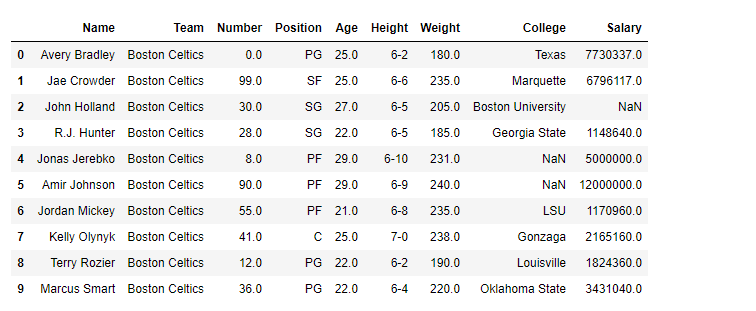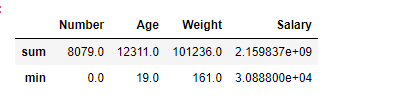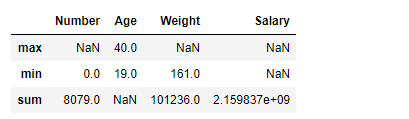Open in App
Not now

# Python | Pandas dataframe.aggregate()

• Difficulty Level : Easy
• Last Updated : 15 Jul, 2022

Python is a great language for doing data analysis, primarily because of the fantastic ecosystem of data-centric Python packages. Pandas is one of those packages and makes importing and analyzing data much easier.

Dataframe.aggregate() function is used to apply some aggregation across one or more column. Aggregate using callable, string, dict, or list of string/callables. Most frequently used aggregations are:

sum: Return the sum of the values for the requested axis
min: Return the minimum of the values for the requested axis
max: Return the maximum of the values for the requested axis

Syntax: DataFrame.aggregate(func, axis=0, *args, **kwargs)

Parameters:
func : callable, string, dictionary, or list of string/callables. Function to use for aggregating the data. If a function, must either work when passed a DataFrame or when passed to DataFrame.apply. For a DataFrame, can pass a dict, if the keys are DataFrame column names.
axis : (default 0) {0 or ‘index’, 1 or ‘columns’} 0 or ‘index’: apply function to each column. 1 or ‘columns’: apply function to each row.

Returns: Aggregated DataFrame

Example #1: Aggregate ‘sum’ and ‘min’ function across all the columns in data frame.

 `# importing pandas package``import` `pandas as pd`` ` `# making data frame from csv file``df ``=` `pd.read_csv(``"nba.csv"``)`` ` `# printing the first 10 rows of the dataframe``df[:``10``]`Aggregation works with only numeric type columns.

 `# Applying aggregation across all the columns ``# sum and min will be found for each ``# numeric type column in df dataframe`` ` `df.aggregate([``'sum'``, ``'min'``])`

Output:
For each column which are having numeric values, minimum and sum of all values has been found. For dataframe df , we have four such columns Number, Age, Weight, Salary.Example #2:

In Pandas, we can also apply different aggregation functions across different columns. For that, we need to pass a dictionary with key containing the column names and values containing the list of aggregation functions for any specific column.

 `# importing pandas package``import` `pandas as pd`` ` `# making data frame from csv file``df ``=` `pd.read_csv(``"nba.csv"``)`` ` `# We are going to find aggregation for these columns``df.aggregate({``"Number"``:[``'sum'``, ``'min'``],``              ``"Age"``:[``'max'``, ``'min'``],``              ``"Weight"``:[``'min'``, ``'sum'``], ``              ``"Salary"``:[``'sum'``]})`

Output:
Separate aggregation has been applied to each column, if any specific aggregation is not applied on a column then it has NaN value corresponding to it.My Personal Notes arrow_drop_up